# RichInStyle.com - Proposals: Floats, an alternative perspective

## Rationale

Although the current CSS float specification provides an excellent floats model, it suffers from flaws of expression, namely:

1. that it is difficult to understand;
2. that it contains errors;
3. that it is not structured in a logical way, which tends to contribute to both of the above.

These problems mean that the specification is likely to be poorly understood, a concern evinced by the state of support in all browsers released to date - I feel that part of the reason for the low quality of most implementations to date is that people have not understood what the specification is saying.

Moreover, I feel that this section of the specification's difficult nature has led to a very poor understanding of floats among page authors, with the number of people who truly understand it numbering only a handful; for example, an erroneous float example was cited on the CIWAS newsgroup, which is generally populated by people who have a better understanding of CSS than most, and no-one pointed out that it was incorrect. This I believe demonstrates the need for a clearer spec on floats.

As a result, I have produced this document. It is essentially a restatement of the current position, removing errors and ambiguities, and although it does include some changes (clearly noted as such), it is principally a restatement.

## Introduction to floats

Floats are used to create the appearance of elements flowing around one another. They can be used in three main ways:

• To create effects with text flowing around content; e.g., drop caps.
• To ensure that inline text is aligned with the left or right of its block.
• To have block elements side-by-side; e.g., to achieve column effects.

## How are these effects achieved?

The basic principle of floats is that they are withdrawn from the normal flow of the document, and do not affect the position of subsequent block elements. However, inline elements, including line boxes, do take the position of floats into account - they flow around them.

As a result, although floats overlap with block elements, they do not overlap with their inline content - it is displaced to one side. In addition, although block elements do not take into account previous floats, floats themselves do take into account previous block elements, as well as the horizontal (but not vertical position) of previous floats.

In addition, floats are moved as far to the left (if ```float: left```) or right (if `float: right`) as possible; and when floats were originally inline, they are aligned with the top of the line box from which they were displaced.

In a nutshell floats can be summarized thus:

1. floating an element causes subsequent non-floating block elements to overlap with it, with their inline content flowing around the float, and
2. floating an element causes subsequent floating elements to flow next to the float.

Here are some examples of the main effects and the code used to achieve it:

DIV.column1 {float: left;
width: 50%}
DIV.column2 {float: left;
width: 50%}

<DIV class="column1">
<a href="#">MP3</a>
<a href="#">CDs</a>
<a href="#">DVD</a>
</DIV>
<DIV class="column2">
<a href="#">Account details</a>
</DIV><P>
<SPAN style="float: left; font-size: 3em; line-height: 3em">T</span>he newspaper article with a dropcap.
</P>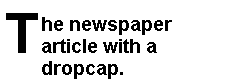### Borders and backgrounds

It is generally the rule that later elements in CSS overlap earlier ones. Floats are one of the two exceptions to this general rule (the other being elements with z-index). Floats are always rendered on top of block elements. Since inline content is displaced to the side of floats, it is rarely the case that it will overlap with block elements; however, when it does (e.g., because of a negative margin), it will be rendered on top of the float.

The importance of this is that it means that the backgrounds and borders of elements that overlap with floats (as will happen when a float is followed by a non-floating block element) will be rendered behind them.

### The width of floated elements

Block-level non-replaced floats (e.g., P, DIV, etc.) must be assigned an explicit width, since otherwise the float could equally well be rendered as ``` width: 100%```, `width: 50%`, etc.

This requirement does not apply to inline-level non-replaced  or replaced elements, since in the former case the width of the content provides the width of the element, and in the latter case if `width` is omitted, its value is determined by the intrinsic value for the element.

Those wishing to extend the width of floating inline-level non-replaced elements (as in:

<P>
<SPAN style="float: left; width: 3em; line-height: 5em; font-size: 6em">A</SPAN> drop cap
</P>

) should note that although width is not valid on inline boxes, it is valid on inline-level block boxes (i.e., floated inline-level elements) (since they are then, as with all floats, block boxes), but its use is not recommended, since there is no way of determining the width of a glyph without access to detailed information about the font. For example, width: 1em might look sensible given 'm', but less so given 'i'. As a result, you are advised to rely on width: auto, which would assign to the element the width that is appropriate, and then add or remove space using negative or positive padding and margins.

Note that unless the inline-level float is assigned a width, it will never wrap onto another line, so for floats that contain a lot of content, it is recommended that a width is assigned, which will cause the element to wrap at 'width'.

### What if a floated element is wider than its containing block?

If an element is flowing next to another float, and will overflow its containing block, it will be moved below that float and then rendered there.

For example:

<DIV style="float: left; width: 50%">
<DIV style="float: left; width: 60%">

This would result in the second DIV being moved below the first because it would otherwise overflow the containing block.

Equally:

<DIV style="float: left; width: 50%">
<DIV style="float: left; width: 110%">

In this case the element would be moved just below the first, regardless of the fact that it will still overflow - the UA must always minimize overflow, and it will then overflow the containing block.

If, as in this case, the floated element is still wider than its containing block, it will overflow the containing block in the normal manner.

Although this method caters for generated pages, where it might be impossible to predict whether the element will overflow the containing block (e.g., where you have the results of a search query floated side by side and you do not know which data will result from a query, and hence cannot say DIV.datum6 {clear: left}, DIV.datum11 {clear: left}, etc.), you might wish to have elements that do overflow their containing block.

To cater for this, CSS provides the block-overflow: float option , so

DIV.column1 {width: 45%;
height: 200px;
background: green;
border: red solid;
float: left}
DIV.column2 {width: 60%;
background: blue;
border: black solid;
height: 200px;
float: right;
block-overflow: float}
<DIV class="column1">
</DIV>
<DIV class="column2">
</DIV>

Would be rendered thus: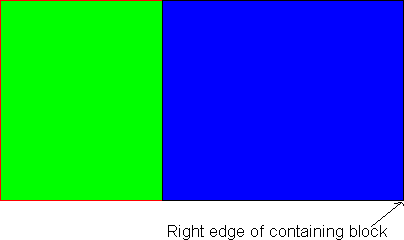Note the float: right declaration. The right of the containing block (and hence the right of the right-floated element) is 100% of the way across the block, and thus floating to the right means that the left edge of the float is just over 40% across (i.e., 100% - 60% (width) - border width). However, since this would result in overlap with the previous column, the normal result would be to move the right-floated element down below the float. The effect of block-overflow: float is to waive this, thus causing overlap.

Compare that with:

DIV.column1 {width: 45%;
background: green;
border: red solid;
height: 200px;
float: left}
DIV.column2 {width: 60%;
background: blue;
border: black solid;
height: 200px;
float: left;
block-overflow: float}

Which would be rendered thus: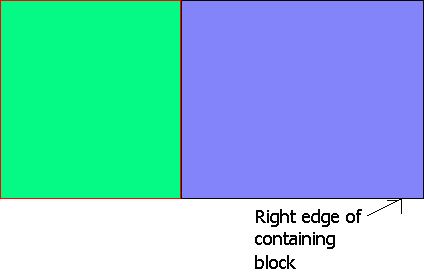In this case, the normal result would be to place the left margin edge of column2 next to the right margin edge of any previous float, which is 45% of the way across the containing block. The right edge would be placed 45% + total width (60%) + border width = about 105% of the way across. Since 105% is greater than 100% (i.e., the width of the containing block), normally this would result in the element being moved down below the float, but block-overflow: float waives this, and the containing block is overflown.

## Examples

### Block examples

These illustrate the general principles of floats:

### Example 1

DIV.float {border: solid red;
background: green;
float: left;
height: 100px;
width: 200px}
DIV.notfloating {border: solid black;
background: blue;
width: 400px;
line-height: 12px}
<DIV class="float">
</DIV>
<DIV class="notfloating">
Some text in the non-floating elemen that will wrap around the floating element. Notice how the line height is observed - the line spacing remains constant - the only thing that changes is the height of the line box.
</DIV>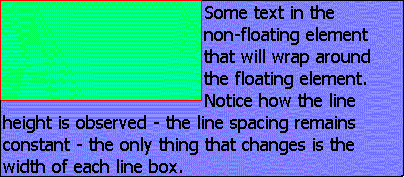You can see in this example that subsequent block elements to a float do not take into account the position of that float, but inline content does take into account the float, being displaced by it. In addition, you can see that the block element's border is obscured by that of the float, since it is generally the rule that subsequent elements are drawn over previous ones. In addition, one thing is particularly noteworthy:

• Even though the float only fully occupies 8 line boxes (each has a height of 12px), it has the effect of shortening 9, since you cannot shorten only half of the ninth, so you must shorten the whole thing.

### Example 2

DIV.float {border: solid red;
float: left;
height: 100px;
width: 500px;}
DIV.notfloating {border: solid green;
width: 400px;
line-height: 12px;}
<DIV class="float">
</DIV>
<DIV class="notfloating">
Some text in the non-floating element.
</DIV>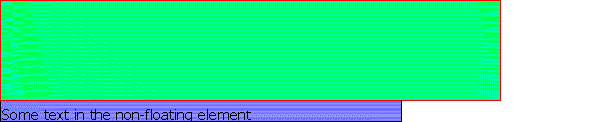In this example, even though the previous float is wider than the block element, the line boxes still exist (but are 0 pixels wide and thus invisible), and hence the inline content (which is displaced by floats) starts in the tenth line box down (since the first line box that is not shortened to zero by the float is the tenth - 100 / 12 is 8.33; if part of a line box is overlapped, the whole must be - you cannot just reduce the line box height - thus meaning nine line boxes are shortened to zero by the float), which means that the float appears to have a 8 pixel margin-bottom.

### Example 3

DIV.float {border: solid red;
float: left;
height: 100px;
width: 400px;}
DIV.notfloating {border: solid green;
width: 400px;
overflow: hidden;
height: 115px;
line-height: 12px}
<DIV class="float">
</DIV>
<DIV class="notfloating">
Some text in the non-floating element.
</DIV>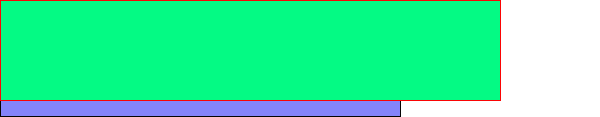In this example, since the non-floating element is not affected by the floating element, it exactly overlaps with it. However, since inline content is displaced by floats, and since ``` overflow``` is set to `hidden` (meaning that the element cannot be increased in height to allow for the content), there is nowhere for the inline content ('Some text in the non-floating element') to be displaced to (since the line boxes are rendered at 0-12px (0 pixels wide), 13-24px (0 pixels wide), 25-36px (0 pixels wide), 37-48px (0 pixels wide), 49-60px (0 pixels wide), 61-72px (0 pixels wide), 73-84px (0 pixels wide), 85-96px (0 pixels wide), and 97-108px (0 pixels wide - some of the float overlaps to this level, and hence the whole line box is reduced by the width of that float), with 109-115px effectively padding (since the line box is 12px high, it requires from 109-120px)), and thus it cannot be rendered.

### Example 4

DIV.float1 {border: solid blue;
float: left;
width: 45%;
height: 200px}
DIV.nonfloat {border: solid red;
height: 100px;}
DIV.float2 {border: solid green;
width: 45%;
height: 300px;
float: left}
<DIV class="float1">
</DIV>
<DIV class="nonfloat">
</DIV>
<DIV class="float2">
</DIV>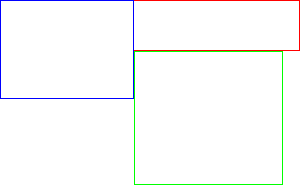This example shows how floats take into the vertical position of previous block elements but not of previous floated elements, whose horizontal position only is taken into account.

In addition, it shows that a subsequent float continues in the vertical line that it was forced into by a float, even though that float might not be as high as it is.

### Example 5

DIV.float1 {border: solid red;
float: left;
width: 45%;
height: 200px}
DIV.nonfloat {border: solid blue;
height: 100px}
DIV.float2 {border: solid green;
width: 45%;
height: 300px;
float: right}
<DIV class="float1">
</DIV>
<DIV class="nonfloat">
</DIV>
<DIV class="float2">
</DIV>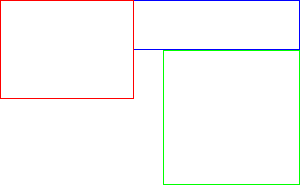This example shows the difference between ```float: left``` and `float: right` - with the previous example, the 'spare' 10% (minus the space taken up by the borders) of space was placed to the right of the second element, but in this case it is placed between them, since one is aligned with the left of the containing block and the other with its right.

### Example 6

DIV.float1 {border: solid blue;
float: left;
width: 45%;
height: 200px}
DIV.nonfloat {border: solid red;
height: 100px;}
DIV.float2 {border: solid green;
width: 60%;
height: 300px;
float: left}
<DIV class="float1">
</DIV>
<DIV class="nonfloat">
</DIV>
<DIV class="float2">
</DIV>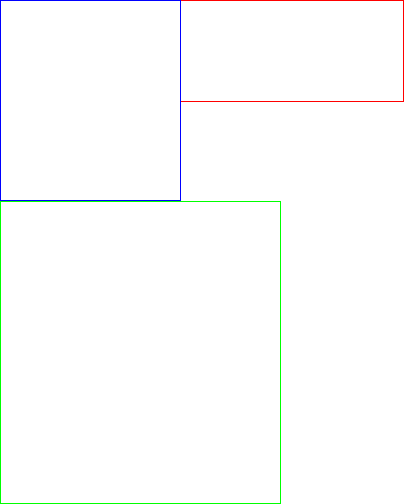This example shows that where an element is so wide that it will overflow its containing block, it is moved below any float (note in this case that the result would have been the same given `float: right`).

### Example 7

DIV.float {float: left;
background: blue;
height: 100px;
width: 400px}
DIV.container {width: 500px}
DIV.nonfloat {background: red;
height: 300px}
<DIV class="container">
<DIV class="float">
</DIV>
<DIV class="nonfloat">
</DIV>
</DIV>In this example, the background of the non-floating element covers all of the area of the containing block, except for the 400px by 100px where the float (recall that floats are rendered in front of non-floating block elements) overlaps with it.

### Example 8

DIV {color: black;
background: white}
DIV.float {float: left;
height: 100px;
width: 400px}
IMG.nonfloat {width: 300px}
DIV.container {width: 600px}
<DIV class="container">
<DIV class="float">
</DIV>
<IMG class="nonfloat">
</DIV>

In this example, since there is not enough room for the replaced element, it is moved below the float, and hence the height of DIV.container is 400 pixels (300 pixels for the image, plus 100 pixels since it must start below the float).

### Inline examples

When an element to be floated is initially inline, it is moved to the left or right until it hits either the outer edge of a previous float or the content edge of the containing block.

Thus, even though in

<P>
Some text <SPAN style="float: left">here</SPAN>
</P>

'here' occurs after 'Some text', the result would be:

hereSome text

Note that there is no space between the final 'e' of 'here' and the 'S' of 'Some'. This is because width: auto results in the element being given the width that its content requires. You should also note that all text on that line is re-flowed to compensate.

If the current line is not wide enough to fit the float as a result of an earlier float on that line (bear in mind that inline floats are moved to the left (or right) until they hit an earlier float or the content edge of the containing block), the element is moved down to the first float-free line. For example:

<P style="width: 200px; line-height: 20px;">
<IMG id="one" style="width: 100px; float: left; height: 105px"> <IMG style="width: 150px; float: left; height: 100px" id="two">Some textual content here
</P>

In this case image one would be floated as per normal. This would align its top with the top of the line box from which it was displaced.

Since it is 105 pixels high, and since each line box is 20 pixels high (because of the `line-height` declaration), the first 6 line boxes would be shortened by the width of the IMG, and thus these six would be only 100 pixels wide instead of the normal 200 pixels. It can be seen that even though the element does not exactly cover the final line box, it is effectively given a `margin-bottom` of 15 pixels, since you can't shorten only part of a line box.

In addition, since image two is wider than the available space in the line box that the markup suggests, it must be moved down. Since inline floats must be aligned with the top of a line box, the float will be moved down to the first line box that is at least as wide as the float. In this case, that is the seventh line box, so the element is aligned with the top of the seventh line box, and since the element is 100px high, the seventh through eleventh line boxes (100px = 5 line boxes at 20px each), will be shortened by the width of the element, thus making them 50px wide.

However, since floats do not affect the dimensions of block elements except insofar as the re-flow of inline content is caused, the height of the P element would only be 140 or 160 pixels (depending on whether 'Some textual content here' took up one line or two), i.e., since 'Some textual content here' must follow image two, and since 'Some textual content here' is part of the block element (<P>), the height of the block must be that of the number of line boxes that 'Some textual content' takes up, plus the number of line boxes that must precede it.

<P style="width: 200px; line-height: 20px;">
<IMG id="one" style="width: 100px; float: left; height: 105px"> <IMG style="width: 250px; float: left; height: 100px" id="two">Some textual content here
</P>

In this case image two will again be aligned with the top of the seventh line box, but in this case since the width of the containing block is 200 pixels, and since the maximum width of a line box is that of the containing block, each of line boxes 7-11 are 0 pixels wide, but still 20 pixels high. As a result, 'Some textual content here' (since it must follow image two) must appear on line box 12, and thus the height of the containing block is 240 pixels (= 20 * 12).

<P style="width: 200px; line-height: 20px;">
<IMG id="one" style="width: 100px; float: left; height: 105px"> <IMG style="width: 250px; float: left; height: 100px" id="two">
</P>

The result here would be a 0 pixel high P element, since the height of a static block element without block-level children is from the top of the top line box to the bottom of the bottom non-empty line box , and thus its height is 0.

### Floated elements assigned a width

If inline non-replaced elements are floated, because they are inline blocks, their width is normally determined by the width of their content; however, if they are explicitly assigned a width this will not be so.

The effect of setting width on inline floats is thus:

• The element behaves like a block element, with content wrapping onto a new line when it reaches the end of one line. For example:

<P style="width: 600px; overflow: hidden">
<SPAN style="width: 200px; float: left">A long line of text that would, with ``` display: block``` and `width: 200px`, have its surplus content chopped off, or, with `width: auto`, the overflow of the containing block.</SPAN>
</P>

Would result in something like this:

A long line of text that would,
with display: block and width:
200px, have its surplus content
chopped off, or, with width:
auto, the overflow of the
containing block.

### Positioning of the edges of floated and non-floated elements

These are the rules that define floats. They are the same in effect as CSS2's rules, but should be easier to implement. They define every element in terms of the edges of blocks.

#### Block-level elements 

##### Vertical positioning
###### `Clear: none`
1. The top margin edge of these elements is aligned with the bottom margin edge of the previous non-floated block-level element.
###### `Clear: left`
1. The top margin edge of these elements is aligned with the bottom margin edge of the previous non-right-floated block-level element (i.e., with the bottom of the previous non-floated or left-floated block-level element).
###### `Clear: right`
1. The top margin edge of these elements is aligned with the bottom margin edge of the previous non-left-floated block-level element.
###### `Clear: both`
1. The top margin edge of these elements is aligned with the bottom margin edge of the previous block-box element (i.e., including floated elements, which are block boxes).
##### Horizontal edges
###### `Float: none`
1. The left margin edge of these elements is aligned with the left content edge of the containing block, provided ```direction: ltr```. The right margin edge is deduced from this (i.e., using width, margins, etc.).
2. If `direction: rtl`, then the right margin edge of these elements is aligned with the right content edge of the containing block. The left margin edge is deduced from this (i.e., using width, margins, etc.).
###### `Float: left`
1. If the top margin edge (determined under Vertical Positioning) of the float is above the bottom margin edge of a previous float element (i.e., if the float is 'current'), the left margin edge of the float is aligned with the right margin edge of that previous float.
2. Otherwise the left margin edge of the float is aligned with the left content edge of its containing block.
3. The position of the right margin edge is then determined in the normal manner (i.e., using width [required], margins, borders and padding).
4. If `block-overflow` is `normal`, then if the float's right margin edge is to the right of the right inner edge of the containing block and a float is 'current', or if the float's right margin edge is to the right of the left margin edge of any 'current' float, the element's top margin edge is realigned as with the next block-level (i.e., including floated) element following the one that it is currently aligned with, and the process re-starts at step 1 .
5. If `block-overflow` is set to float, then the element is rendered where it is, causing overlap with other floats and/or overflow of the containing block.
###### `Float: right`
1. If the top margin edge of the float is above the bottom margin edge of a previous float (i.e., if the float is 'current'), the right margin edge of the float is aligned with the right margin edge of that previous float.
2. Otherwise the right margin edge of the float is aligned with the right content edge of the containing block.
3. The position of the left margin edge is then deduced from this; e.g., with a total width of 60%, and a right edge aligned with that of the containing block, the left edge would be 40% across the containing block.
4. If `block-overflow` is `normal`, then if the float's left margin edge is to the left of the left content edge of the containing block and a float is 'current', or if the float's left margin edge is to the left of the right margin edge of any 'current' float, the element's top margin edge is realigned as with the next block-level (i.e., including floated) element following the one that it is currently aligned with, and the process re-starts at step 1.
5. If `block-overflow` is set to float, then the element is rendered where it is, causing overlap with other floats and/or overflow of the containing block.

#### Inline elements

##### Vertical positioning
###### `Float: left/right`
1. The top margin edge of these elements is aligned with the top of the line box from which they were displaced.
2. The bottom margin edge is determined in the usual manner; i.e., by using or calculating `height`, margin, border and padding. If this does not result in the bottom of the float being aligned with the bottom of a line box, margin-bottom is increased so that this occurs.
###### Line boxes (i.e., `float: none`)
1. The top edge of a line box is aligned with the bottom of the previous line box in that block, unless there is no previous line box, in which case it is the top content edge of the containing block.
2. The distance to the bottom edge of a line box is determined by the rules for calculating the height of line boxes.
##### Horizontal edges
###### `Float: left`
1. If the top margin edge of the float is above (determined under vertical positioning) the bottom margin edge of a previous float (i.e., if the float is 'current'), the left margin edge of the float is aligned with the right margin edge of that previous float.
2. Otherwise the left margin edge of the float is aligned with the left content edge of its containing block.
3. The position of the right margin edge is then determined in the normal manner (i.e., using width (required if ```display: inline-block```; if not specified then equal to the content width), margins, borders and padding).
4. If `block-overflow` is `normal`, then if the float's right margin edge is to the right of the right inner edge of the containing block and a float is 'current', or if the float's right margin edge is to the right of the left margin edge of any 'current' float, the element's top margin edge is realigned as with the top of the first line box following the one that it is currently aligned with, and the process re-starts at step 1.
5. If `block-overflow` is set to `float`, then the element is rendered where it is, causing overlap with other floats and/or overflow of the containing block.
###### `Float: right`
1. If the top margin edge of the float is above (determined under Vertical Positioning) the bottom margin edge of a previous float (i.e., if the float is 'current'), the right margin edge of the float is aligned with the right margin edge of that previous float.
2. Otherwise the right margin edge of the float is aligned with the right content edge of the containing block.
3. The position of the left margin edge is then deduced from this, e.g., with a total width of 60%, and a right edge aligned with that of the containing block, the left edge would be 40% across the containing block.
4. If `block-overflow` is `normal`, then if the float's left margin edge is to the left of the left content edge of the containing block and a float is 'current', or if the float's left margin edge is to the left of the right margin edge of any 'current' float, the element's top margin edge is realigned as with the top of the first line box following the one that it is currently aligned with, and the process re-starts at step 1.
5. If `block-overflow` is set to `float`, then the element is rendered where it is, causing overlap with other floats and/or overflow of the containing block.
###### Line boxes (i.e., `float: none`)
1. The left edge of the line box is aligned with the left content edge of the containing block.
2. If that point is to the left of the right margin edge of a float, the left edge is realigned with the right margin edge of that float.
3. The right edge of the line box is aligned with the left margin edge of the next float to its right. If there is no such float, it is aligned with the right content edge of the containing block.
4. If the right edge of the line box has been aligned with the left of a float, then if the right margin edge of that float is not to the right of the right content edge of the containing block, a second line box is formed  with its left margin edge at the right margin edge of that float. The right margin edge is then determined according to rule 3 and the process is repeated until the right edge of a line box has been aligned with the right of the containing block .

## Incidental amendments

### An incidental amendment to overflow

CSS should say for `overflow: auto` 'This should be treated as `overflow: visible`, except on the root element where with `direction: ltr` it is treated as `overflow: scroll` on the root element's right inner edge and `overflow: hidden` on its left content edge. Where `direction: rtl` on the root element, it is treated as `overflow: visible` on its left content edge, and `overflow: hidden` on its right content edge.' [8, 9]

This is to cater for the situation where an element overflows the left edge of the containing block (e.g., float: right; width: 105%).

### Text-align modification

This applies to the content of inline-block elements and to table cell elements.

### Footnotes

1. Not the current position in CSS, but an undoubted improvement; for example, when producing the navigation tables that this site uses, I would have preferred to have used floats. The idea was to have a black background with white text on top with each of the links taking as much space as they required, plus padding-right to add a little separation to each. What I would have liked to do is to have <DIV style="background: black"> <SPAN style="float: left; width: auto; padding-right: 1em"><A href="whatever.html"></A></SPAN> <SPAN style="float: left; width: auto; padding-right: 1em"><A href="whatever.html"></A></SPAN>. Unfortunately, this wouldn't work, so I was forced, not by buggy browsers, but by the CSS specification itself, to use a TABLE for a purpose for which they are not intended.
2. Not the current position in CSS, but an improvement - it should be possible to have floats that overflow the containing block. `block-overflow` is used instead of overflow because of backward-compatibility issues (e.g., overflow: float scroll might be wanted, but should break old implementations).
3. A change from CSS 2, which states that the height of a block element is from the top of the top line box to the bottom of the bottom one. It is a necessary change (i.e., CSS 2 was wrong), since otherwise a float can never go outside its block, as illustrated in the example in CSS 2, where an image is shown floating in one element that continues into the next, as compared with when `clear: left`. My approach is necessary, since the line boxes are still needed for alignment purposes, but will only be allocated if inline content follows an inline float.
4. These rules open the door to position: float, since they are very similar to `left`, ``` right```, etc.
5. This will still result in overflow if the total width exceeds the `width` of the containing block.
6. This removes the ambiguity from CSS 2 whereby the rendering of the following wasn't defined:
7. This will always occur, even if there is a float flush with the right edge of the containing block - this is the reason for using 'not to the right of' rather than to the left of - so that in this case a 0-width line box is formed.
8. This is not an issue to specific to floats, but a general omission from CSS; I think that the behaviour explicated here is that that is expected.
9. It is arguable that elements overflowing the root element's left margin edge with ltr, and its right margin edge with rtl should also extend the root element, but I think that this is wrong.

## Glossary

inline-level and block-level
refer to the formatting context that the element is in; for example, in <p><img style="float: left">, the (block) image is participating in the inline-level formatting context
inline and block
refer to the type of box formed by the element; for example, floats are always block boxes